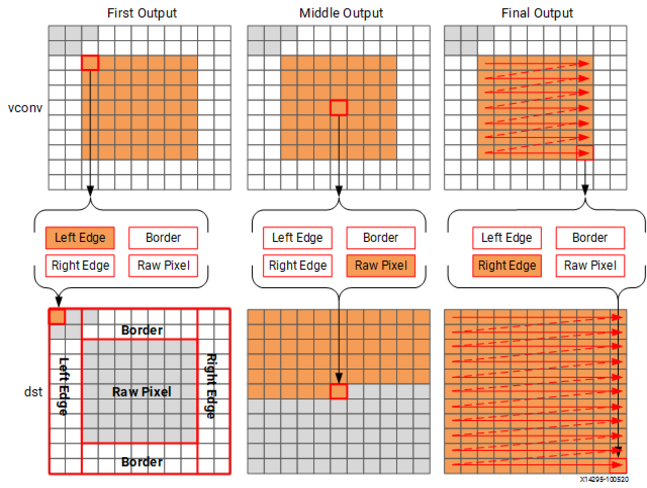# Border Pixels - 2022.1 English

## Vitis High-Level Synthesis User Guide (UG1399)

Document ID
UG1399
Release Date
2022-06-07
Version
2022.1 English

The final step in the algorithm is to replicate the edge pixels into the border region. Once again, to ensure the constant flow or data and data reuse the algorithm makes use of an `hls::stream` and caching.

The following figure shows how the border samples are aligned into the image.

• Each sample is read from the `vconv` output from the vertical convolution.
• The sample is then cached as one of four possible pixel types.
• The sample is then written to the output stream.
Figure 1. Streaming Border SamplesThe code for determining the location of the border pixels is:

``````Border:for (int i = 0; i < height; i++) {
for (int j = 0; j < width; j++) {
T pix_in, l_edge_pix, r_edge_pix, pix_out;
#pragma HLS PIPELINE
if (i == 0 || (i > border_width && i < height - border_width)) {
if (j < width - (K - 1)) {
borderbuf[j] = pix_in;
}
if (j == 0) {
l_edge_pix = pix_in;
}
if (j == width - K) {
r_edge_pix = pix_in;
}
}
if (j <= border_width) {
pix_out = l_edge_pix;
} else if (j >= width - border_width - 1) {
pix_out = r_edge_pix;
} else {
pix_out = borderbuf[j - border_width];
}
dst << pix_out;
}
}
}
``````

A notable difference with this new code is the extensive use of conditionals inside the tasks. This allows the task, once it is pipelined, to continuously process data and the result of the conditionals does not impact the execution of the pipeline: the result will impact the output values but the pipeline with keep processing so long as input samples are available.

The final code for this FPGA-friendly algorithm has the following optimization directives used.

``````template<typename T, int K>
static void convolution_strm(
int width,
int height,
hls::stream<T> &src,
hls::stream<T> &dst,
const T *hcoeff,
const T *vcoeff)
{
#pragma HLS DATAFLOW
#pragma HLS ARRAY_PARTITION variable=linebuf dim=1 type=complete

hls::stream<T> hconv("hconv");
hls::stream<T> vconv("vconv");
// These assertions let HLS know the upper bounds of loops
assert(height < MAX_IMG_ROWS);
assert(width < MAX_IMG_COLS);
assert(vconv_xlim < MAX_IMG_COLS - (K - 1));

// Horizontal convolution
HConvH:for(int col = 0; col < height; col++) {
HConvW:for(int row = 0; row < width; row++) {
#pragma HLS PIPELINE
HConv:for(int i = 0; i < K; i++) {
}
}
}
// Vertical convolution
VConvH:for(int col = 0; col < height; col++) {
VConvW:for(int row = 0; row < vconv_xlim; row++) {
#pragma HLS PIPELINE
#pragma HLS DEPENDENCE variable=linebuf type=inter dependent=false
VConv:for(int i = 0; i < K; i++) {
}
}

Border:for (int i = 0; i < height; i++) {
for (int j = 0; j < width; j++) {
#pragma HLS PIPELINE
}
}
``````

Each of the tasks are pipelined at the sample level. The line buffer is full partitioned into registers to ensure there are no read or write limitations due to insufficient block RAM ports. The line buffer also requires a dependence directive. All of the tasks execute in a dataflow region which will ensure the tasks run concurrently. The hls::streams are automatically implemented as FIFOs with 1 element.